# SSC CGL EXAMS 2018 | Quantitative Aptitude Practice Questions (Day-11)

Dear Aspirants, Here we have given the Important SSC Exam 2018 Practice Test Papers. Candidates those who are preparing for SSC 2018 can practice these questions to get more confidence to Crack SSC 2018 Examination.

[WpProQuiz 4257]

1)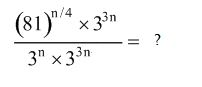a) 1

b) 3

c) 3n

d) None of these

2)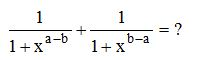a) 0

b) xa + xb

c) 1

d) xa+b

3) Find the number of divisors of 864?

1) 22

2) 24

3) 26

4) None of these

4) If a number is increased by 4 and divided by 8, the result is 6. What would be the result if 2 is added to the number and, then it is divided by 23?

a) 2

b) 1

c) 3

d) None of these

5) The difference between a two-digit number and the number obtained by interchanging the positions of its digits is 18. What is the sum of the two digits of that number?

a) 2

b) 4

c) 6

d) Cannot be determined

6)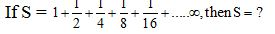a) 4

b) 3/2

c) 2

d) None of these

7) The average temperature on Wednesday, Thursday and Friday was 26o The average temperature on Thursday, Friday and Saturday was 25oC. If the temperature on Saturday was 28oC, what was the temperature (oC) on Wednesday?

a) 29

b) 30

c) 31

d) 32

8) A batsman scored 70 runs in the 50th inning and thus increased his average by a certain number of runs that is a whole number. Which of the following can be a possible value of his new average?

a) 21

b) 22

c) 23

d) All of these

9) Rajeev buys good worth Rs. 6000. He gets a rebate of 10% on it. After getting the rebate, he pays sales tax at the rate of 20%. Find the amount he will have to pay for the goods?

a) Rs. 6,400

b) Rs. 6,480

c) Rs. 6520

d) Rs. 6690

10) Kiran bought two books, which together cost him Rs. 480. He sold one of them at a loss of 15% and other at a gain of 19%. If the selling prices of both the books are equal, find the cost of the lower priced book?

a) Rs. 300

b) Rs. 180

c) Rs. 200

d) Rs. 280

11) After reducing the marked price of a book by Rs. 30, a shopkeeper makes a profit of 40%. If the cost price of the book is Rs. 50, find the profit percentage if the shopkeeper had sold the book at its marked price?

a) 50

b) 70

c) 80

d) 100

12) Find the minimum value of (x – 3) (x – 6)

a) 0

b) 1

c) 2

d) None of these

13) At a dinner party, every two guests used a bowl of fried rice between them, every three guests used a bowl of dal makhani among them and every four guests used a bowl of manchuriyan among them. There are altogether 65 bowls. What is the number of guests present at the party?

a) 90

b) 80

c) 70

d) 60

14) If tan 15o = 2 – , then the value of tan 15o cot 75o + tan 75o cot 15o is

a) 14

b) 12

c) 10

d) 8

15) If x2 + 2 = 2x, then the value of x4 – x3 + x2 + 2 is

a) 0

b) 1

c) –1

d) √2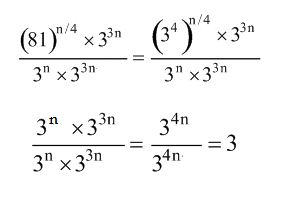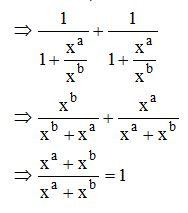864 = 25 × 33

Number of factors = (5+1) (3 + 1)

= 6 * 4 = 24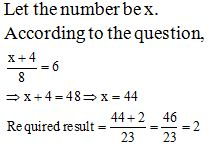13, 24, 35, 46, 57, 68, 79 are the numbers for which difference of the number obtained by interchanging the digits and original number is 18. Sum of digits is different for different numbers.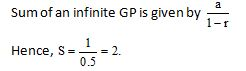Total temperature on Wednesday, Thursday and Friday was 26 3 = 78oC

Total temperature on Thursday, Friday and Saturday was 25  3 = 75oC

Hence, difference between the temperature on Wednesday and Saturday = 3oC

If Saturday temperature = 28oC, then Wednesday’s temperature = 28 + 3 = 31oC

Let his average after 49th inning be n and after scoring 70 runs in the 50th inning.

His average increased by a whole number k.

According to the question.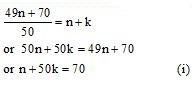Now rest of the work can be done just by checking the option i.e.

Option (1): n + k = 21            (ii)

Solving, we get

n = 20 and k = 1.

This option gives us an integer value of k, hence this one is the correct option.

Similarly, by checking options (b) and (c), we can see that these options do not give us an integer value of k.

Hence, these options are wrong.

Alternative method

Part of runs scored in 50th inning will go toward increasing the average of 49 innings to the new average and the remaining part of the runs will go towards maintaining part of the runs will go towards maintaining the new average for the 40th inning.

The only constraint in this problem is that there is an increase in the average by a whole number of runs. This is possible only for option (1).

Rebate = 10% of 6000 = 600

Sales tax = 20% of (6000 – 600) = 1080

Amount to be paid = 5400 + 1080 = 6480

Solve this question using the options. The first thing you should realize is that the cost of the lower priced book should be less than Rs. 240. Thus, we can reject options (1) and (4). Checking option (3) we can see that if the lower priced book is priced at Rs. 200, the higher priced item would be priced at Rs. 280. Then

1.19 * 200 = 238

and

0.85 * 280 = 238

It can be seen that in this condition the values of the selling price of both the items would be equal (as required by the conditions given in the problem).

Actual selling price = 50 + 40% of 50 = Rs. 70.

Marked price = 70 + 30 = Rs. 100.

Required profit per cent.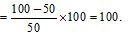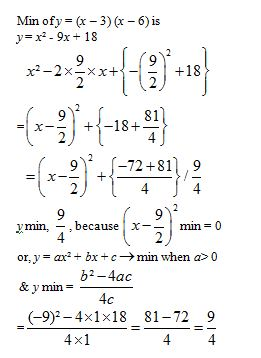Let the number of guests while 2 at a time = x

Let the number of guests while 3 at a time= y

Let the number of guests while 4 at a time = z

x + y + z = 65                …… (i)

We have 2x = 3y = 4z                …… (ii)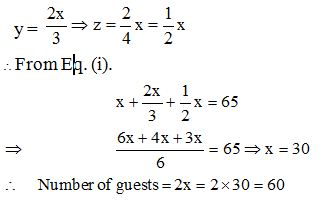Given, tan 15° = 2 – √3

Then, tan 15°. cot 75° + tan 75°.cot 15°

= tan 15°. cot (90° – 15°) + tan (90° – 15°).cot 15°

= tan2 15° + cot2 15°

[tan (90° – q) = cotq, cot (90° – q) = tanq]

Now, tan 15°= 2 – √3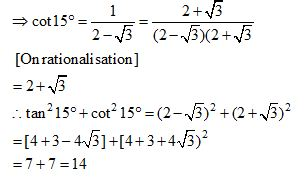Given, x2 + 2 = 2x

x2 – 2x + 2 = 0

Now, x2 – 2x + 2) x4 – x3 + x2 + 2 (x2 + x + 1)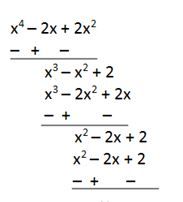Hence, x4 – x3 + x2 + 2 can be represented as = (x2 – 2x + 2) (x2 + x + 1) = 0

### *******************